# Theoretical Computer Science at Boston University

Welcome to the Theoretical Computer Science (TCS) research group. Research in TCS (and in our group) encompasses diverse areas such as theory of computation, complexity, sublinear algorithms, optimization, distributed and parallel computing, data privacy, machine learning and more. The common theme behind the TCS approach to these research directions is a focus on precise models and rigorous mathematical analysis.

We hold a weekly Theory Seminar. For Spring 2023, we meet Mondays from 1:30-2:30pm simultaneously in person and over Zoom. For other events and general announcements, you can subscribe to the TCS mailing list here.

If you are interested in joining the TCS research group, potential PhD students should apply either to the Department of Computer Science or the Faculty of Computing & Data Sciences PhD programs, depending on their interests and intended faculty advisors. Inquiries about postdoc positions should be directed to faculty directly.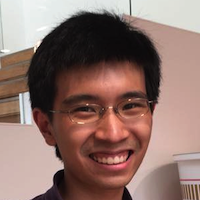Computational Complexity, Data Privacy
Algs & ToCCryptography, Network and System Security, Cybersecurity and Law
Sec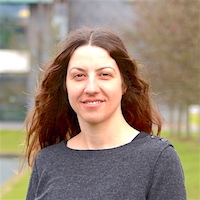Optimization, Algorithms, Machine Learning Theory
Algs & ToCDifferential Privacy, Programming Languages, Formal Methods
PLReliable Computation, Algorithmic Information Theory
Algs & ToCAlgorithmic Mechanism Design & Game Theory, Algorithms, Microeconomics
Algs & ToCAlgorithms
Algs & ToCData Privacy
SecAlgorithms, Probability, Combinatorics
Joining Fall 2024!
Algs & ToCComputational Complexity
Algs & ToC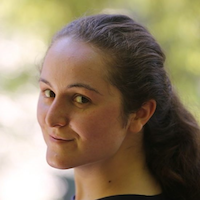Complexity, Average-Case Analysis, Fine-Grained Complexity
Joining Fall 2023!
Algs & ToCAlgorithms for Big Data, Sublinear Algorithms, Streaming
Algs & ToCReinforcement Learning, Bandits, Online Learning
Joining Fall 2024!
MLSublinear Algorithms, Property Testing, Private Data Analysis
Algs & ToCCryptography
SecData Privacy, Machine Learning, Cryptography, Algorithms
Algs & ToC
SecCryptography, Information Security, Distributed-Ledger Technology
Joining Fall 2023!
Sec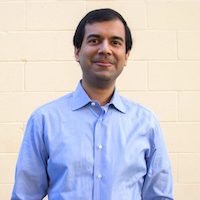Cryptography
SecMachine Learning Theory, Game Theory, Reinforcement Learning
Joining Fall 2023!
MLStatistical Learning Theory, Property Testing, Differential Privacy, Sublinear Algorithms
Algs & ToC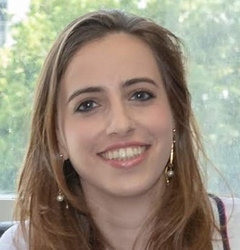Algs & ToCSec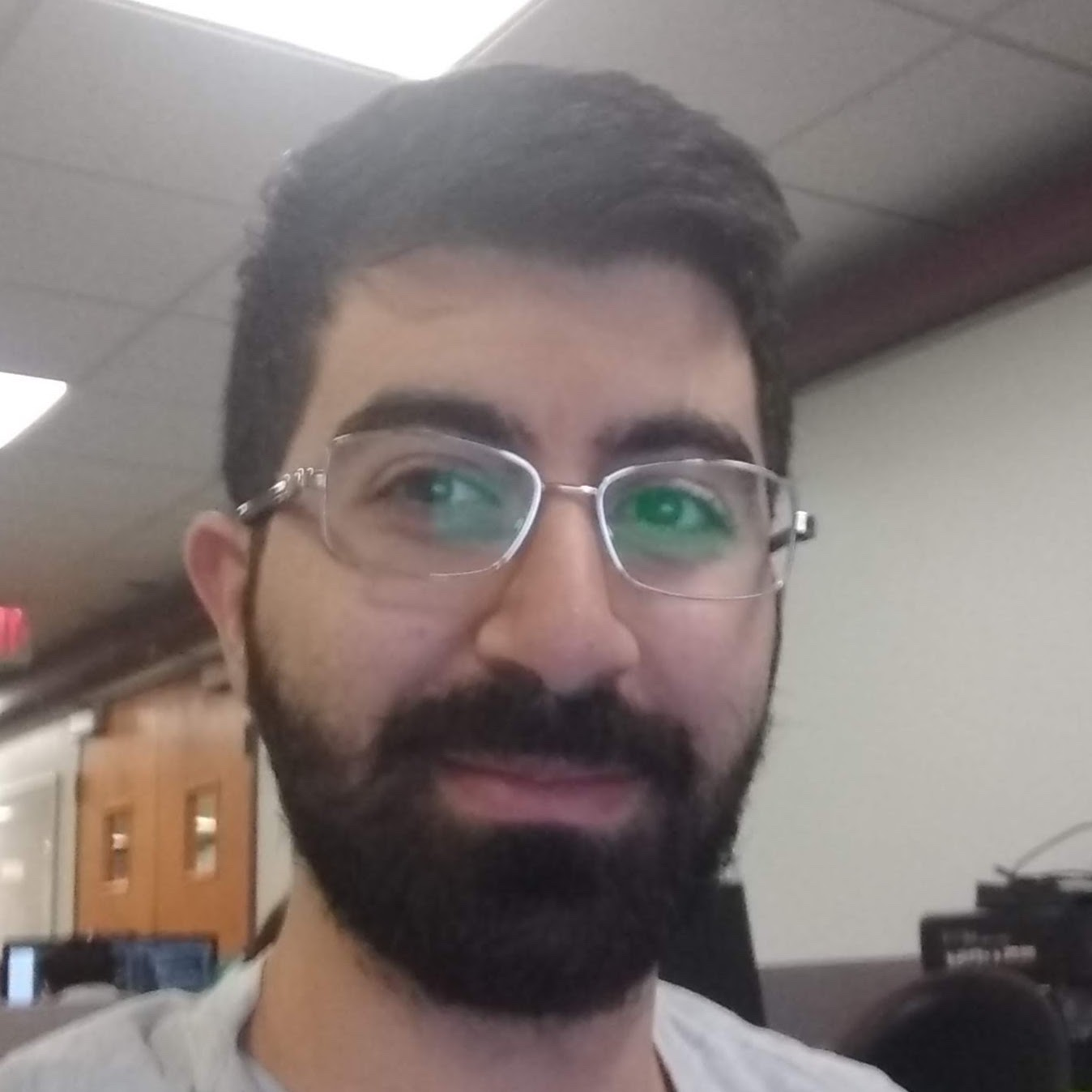SecDifferential Privacy, Machine Learning Theory
Algs & ToC
SecCryptography
SecComplexity Theory and Algorithms
Algs & ToCAlgs & ToCQuantum Computation, Foundations of Computer Science
Algs & ToC
SecDifferential Privacy, Learning Theory
Algs & ToCAlgs & ToC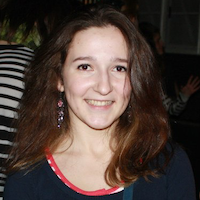Computational Complexity, Differential Privacy, Algorithms
Algs & ToCSecResponsible AI, Privacy, Machine Learning, CS & Law
Algs & ToC
SecComplexity Theory, Fixed Parameter Tractability, Circuit Complexity
Algs & ToCCryptography, Differential Privacy, Privacy Enhancing Technologies
Algs & ToC
SecComplexity theory
Algs & ToCAlgorithms, Privacy
Algs & ToCSecSublinear Algorithms, Property Testing
Algs & ToCSecAlgorithms
PLMachine Learning, Optimization
Algs & ToCAlgs & ToCOptimization, Machine Learning Theory
Algs & ToCQuantum computing, Cryptography
Algs & ToC
SecEconomics and Computation
Algs & ToC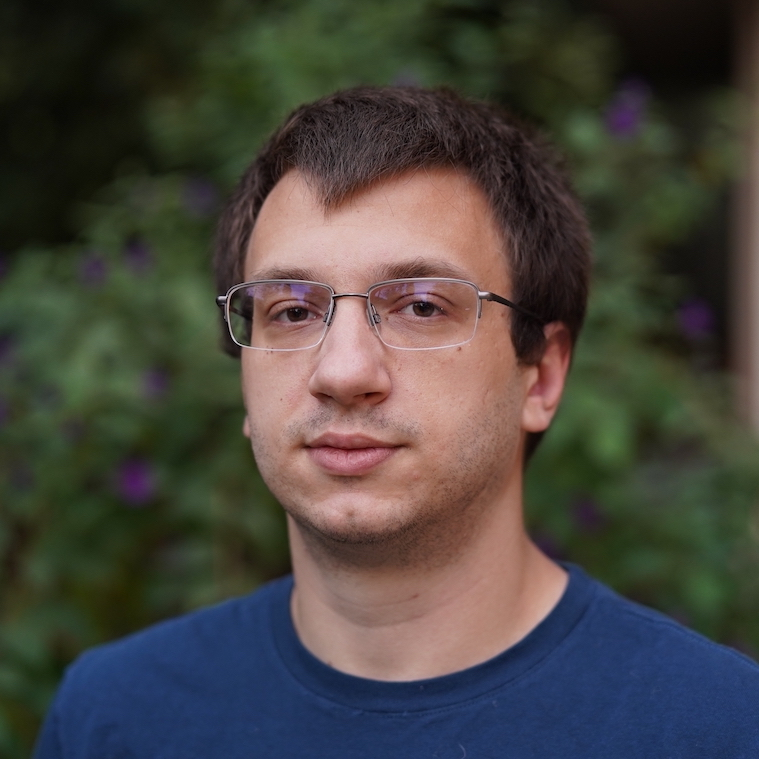Algs & ToCSecTheoretical and Applied Cryptography, Secure Computation, Zero Knowledge
Algs & ToC
SecDifferential Privacy, Adaptive Data Analysis, Learning Theory
Algs & ToC
SecAlgs & ToCTheory and Practice of Data Privacy
SecSublinear Computation, Approximation Polynomials, Quantum Computation
Algs & ToCData Privacy, Algorithms
Algs & ToC
SecSec